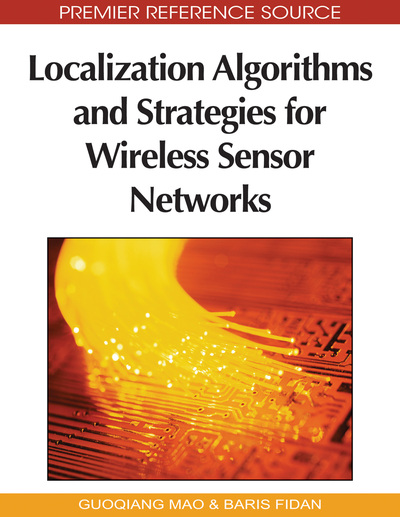# Graph Theoretic Techniques in the Analysis of Uniquely Localizable Sensor Networks

Bill Jackson (University of London, UK) and Tibor Jordán (Eötvös University, Hungary)
DOI: 10.4018/978-1-60566-396-8.ch006

## Abstract

In the network localization problem the goal is to determine the location of all nodes by using only partial information on the pairwise distances (and by computing the exact location of some nodes, called anchors). The network is said to be uniquely localizable if there is a unique set of locations consistent with the given data. Recent results from graph theory and combinatorial rigidity made it possible to characterize uniquely localizable networks in two dimensions. Based on these developments, extensions, related optimization problems, algorithms, and constructions also became tractable. This chapter gives a detailed survey of these new results from the graph theorist’s viewpoint.
Chapter Preview
Top

## Introduction

In the network localization problem the locations of some nodes (called anchors) of a network as well as the distances between some pairs of nodes are known, and the goal is to determine the location of all nodes. This is one of the fundamental algorithmic problems in the theory of wireless sensor networks and has been the focus of a number of recent research articles and survey papers, see for example (Aspnes et al., 2007; Eren et al., 2004; Mao et al., 2007; So & Ye, 2007)

A natural additional question is whether a solution to the localization problem is unique. The network, with the given locations and distances, is said to be uniquely localizable if there is a unique set of locations consistent with the given data. The unique localizability of a two-dimensional network, whose nodes are ‘in generic position’, can be characterized by using results from graph rigidity theory. In this case unique localizability depends only on the combinatorial properties of the network: it is determined completely by the distance graph of the network and the set of anchors, or equivalently, by the grounded graph of the network and the number of anchors. The vertices of the distance and grounded graph correspond to the nodes of the network. In both graphs two vertices are connected by an edge if the corresponding distance is explicitly known. In the grounded graph we have additional edges: all pairs of vertices corresponding to anchor nodes are adjacent. The grounded graph represents all known distances, since the distance between two anchors can be obtained from their locations. Before stating the basic observation about unique localizability we need some additional terminology. It is convenient to investigate localization problems with distance information by using frameworks, the central objects of rigidity theory.

A d-dimensional framework (also called geometric graph or formation) is a pair (G, p), where G = (V,E) is a graph and p is a map from V to Rd. We consider the framework to be a straight line realization of G in Rd. Two frameworks (G, p) and (G, q) are equivalent if corresponding edges have the same lengths, that is, if ||p(u) − p(v)|| = ||q(u) − q(v)|| holds for all pairs u, v with uvE, where ||.|| denotes the Euclidean norm in Rd. Frameworks (G, p), (G, q) are congruent if ||p(u) − p(v)|| = ||q(u) − q(v)|| holds for all pairs u, v with u, vV . This is the same as saying that (G, q) can be obtained from (G, p) by an isometry. We shall say that (G, p) is globally rigid, or that (G, p) is a unique realization of G, if every framework which is equivalent to (G, p) is congruent to (G, p), see Figure 1.

Figure 1.

Two realizations of the same graph G in R2: F1 is globally rigid; F2 is not since we can obtain a realization of G which is equivalent but not congruent to F2 by reflecting p2 in the line through p1, p5, p3.

## Complete Chapter List

Search this Book:
Reset